# Plotting Fractions on a Number Line Online Quiz

#### Complete Python Prime Pack

9 Courses     2 eBooks

#### Artificial Intelligence & Machine Learning Prime Pack

6 Courses     1 eBooks

#### Java Prime Pack

9 Courses     2 eBooks

Following quiz provides Multiple Choice Questions (MCQs) related to Plotting Fractions on a Number Line. You will have to read all the given answers and click over the correct answer. If you are not sure about the answer then you can check the answer using Show Answer button. You can use Next Quiz button to check new set of questions in the quiz.### Explanation

Step 1:

Marks divide each unit into 4 equal parts on number line here.

Step 2:

To plot 1/2 or 2/4, we put a point between 0 and 1, 2 marks to the right of 0.

Step 3:

To plot 1$\frac{2}{4}$, we put a point between 1 and 2, 2 marks to the right of 1.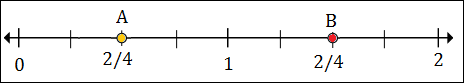### Explanation

Step 1:

Marks divide each unit into 6 equal parts on number line here.

Step 2:

To plot 1$\frac{1}{2}$ or 1$\frac{3}{6}$, we put a point between 1 and 2; 3 marks to the right of 1.

Step 3:

To plot 2$\frac{5}{6}$, we put a point between 2 and 3, 5 marks to the right of 2.### Explanation

Step 1:

Marks divide each unit into 8 equal parts on number line here.

Step 2:

To plot 1$\frac{1}{4}$ or 1$\frac{2}{8}$, we put a point between 1 and 2; 2 marks to the right of 1.

Step 3:

To plot 2$\frac{5}{8}$, we put a point between 2 and 3; 5 marks to the right of 2.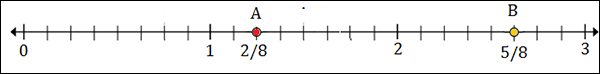### Explanation

Step 1:

Marks divide each unit into 8 equal parts on number line here.

Step 2:

To plot 1$\frac{1}{8}$, we put a point between 1 and 2, 1 mark to the right of 1.

Step 3:

To plot 2$\frac{5}{8}$, we put a point between 2 and 3, 5 marks to the right of 2.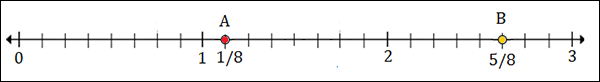### Explanation

Step 1:

Marks divide each unit into 3 equal parts on number line here.

Step 2:

To plot $\frac{1}{3}$, we put a point between 0 and 1, 1 mark to the right of 0.

Step 3:

To plot 1$\frac{1}{3}$, we put a point between 1 and 2, 1 mark to the right of 1.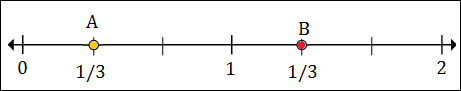### Explanation

Step 1:

Marks divide each unit into 4 equal parts on number line here.

Step 2:

To plot $\frac{3}{4}$, we put a point between 0 and 1, 3 marks to the right of 0.

Step 3:

To plot 1$\frac{1}{2}$ or 1$\frac{2}{4}$, we put a point between 1 and 2, 2 marks to the right of 1.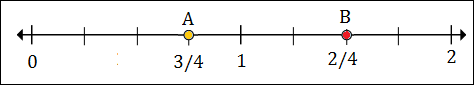### Explanation

Step 1:

Marks divide each unit into 6 equal parts on number line here.

Step 2:

To plot 1$\frac{4}{6}$, we put a point between 1 and 2, 4 marks to the right of 1.

Step 3:

To plot 2$\frac{1}{6}$, we put a point between 2 and 3, 1 mark to the right of 2.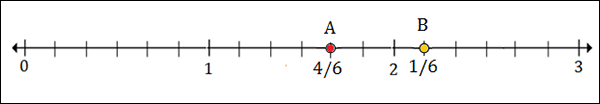### Explanation

Step 1:

Marks divide each unit into 6 equal parts on number line here.

Step 2:

To plot 1$\frac{1}{6}$, we put a point between 1 and 2, 1 mark to the right of 1.

Step 3:

To plot 2$\frac{4}{6}$, we put a point between 2 and 3, 4 marks to the right of 2.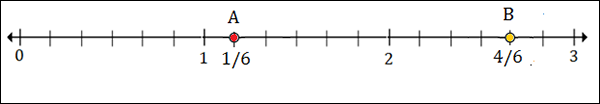### Explanation

Step 1:

Marks divide each unit into 4 equal parts on number line here.

Step 2:

To plot $\frac{1}{2}$ or $\frac{2}{4}$ we put a point between 0 and 1, 2 marks to the right of 0.

Step 3:

To plot 1$\frac{3}{4}$, we put a point between 1 and 2, 3 marks to the right of 1.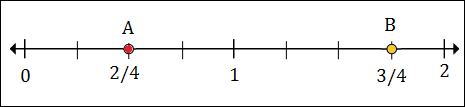### Explanation

Step 1:

Marks divide each unit into 6 equal parts on number line here.

Step 2:

To plot 1$\frac{1}{2}$ or 1$\frac{2}{4}$ we put a point between 1 and 2, 2 marks to the right of 1.

Step 3:

To plot 2$\frac{4}{6}$, we put a point between 2 and 3, 4 marks to the right of 2.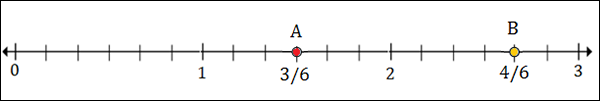plotting_fractions_on_number_line.htm